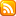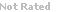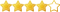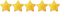Loading... Please wait...

##Featured Products

• R155.97 (inc VAT) R135.63 (ex VAT)• R59.80 (inc VAT) R52.00 (ex VAT)• R49.82 (inc VAT) R43.32 (ex VAT)• R164.89 (inc VAT) R143.38 (ex VAT)• R51.60 (inc VAT) R44.87 (ex VAT)• R122.99 (inc VAT) R106.95 (ex VAT)• R56.35 (inc VAT) R49.00 (ex VAT)• R155.97 (inc VAT) R135.63 (ex VAT)• R35.64 (inc VAT) R30.99 (ex VAT)• R155.97 (inc VAT) R135.63 (ex VAT)• R172.90 (inc VAT) R150.35 (ex VAT)• R160.33 (inc VAT) R139.42 (ex VAT)• R51.68 (inc VAT) R44.94 (ex VAT)• R26.65 (inc VAT) R23.17 (ex VAT)• R155.97 (inc VAT) R135.63 (ex VAT)• R53.38 (inc VAT) R46.42 (ex VAT)• R155.97 (inc VAT) R135.63 (ex VAT)• R174.69 (inc VAT) R151.90 (ex VAT)• R128.34 (inc VAT) R111.60 (ex VAT)• R155.97 (inc VAT) R135.63 (ex VAT)• R47.15 (inc VAT) R41.00 (ex VAT)• R139.04 (inc VAT) R120.90 (ex VAT)• R49.82 (inc VAT) R43.32 (ex VAT)• R64.40 (inc VAT) R56.00 (ex VAT)• R56.95 (inc VAT) R49.52 (ex VAT)• R44.39 (inc VAT) R38.60 (ex VAT)• R133.69 (inc VAT) R116.25 (ex VAT)• R101.20 (inc VAT) R88.00 (ex VAT)• R142.51 (inc VAT) R123.92 (ex VAT)• R139.04 (inc VAT) R120.90 (ex VAT)• R164.89 (inc VAT) R143.38 (ex VAT)• R37.34 (inc VAT) R32.47 (ex VAT)• R132.25 (inc VAT) R115.00 (ex VAT)• R60.51 (inc VAT) R52.62 (ex VAT)• R93.15 (inc VAT) R81.00 (ex VAT)• R128.34 (inc VAT) R111.60 (ex VAT)